# Python Pillow – Flip and Rotate Images

• Last Updated : 16 Oct, 2021

Prerequisites: Pillow

Python Pillow or PIL is the Python library that provides image editing and manipulating features. The Image Module in it provides a number of functions to flip and rotate images. image.transpose() is the function used to rotate and flip images with necessary keywords as parameters.

Syntax:

image.transpose(appropriate keyword)

In the examples given below, we will be exploring all possible rotations using an appropriate keyword.

Image used:## Flipping Images

• Anticlockwise: To flip an image in anti-clockwise direction the keyword that needs to be passed is Image.TRANSPOSE.

Syntax:

img.transpose(Image.TRANSPOSE)

Example:

## Python

 `from` `PIL ``import` `Image`  `img ``=` `Image.``open``(``'geek.jpg'``)` `# flip anti-clockwise``flip_img ``=` `img.transpose(Image.TRANSPOSE)` `flip_img.show()`

Output :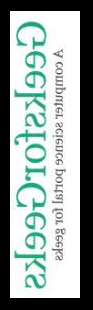Clockwise: To flip an image in the clockwise direction the keyword that needs to be passed is Image.TRANSVERSE.

Syntax:

img.transpose(Image.TRANSVERSE)

Example:

## Python

 `from` `PIL ``import` `Image`  `img  ``=` `Image.``open``(``'geek.jpg'``)` `# flip clockwise``flip_img``=` `img.transpose(Image.TRANSVERSE)` `flip_img.show()`

Output :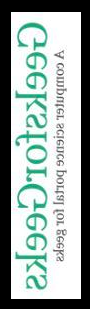Horizontal Flip: For horizontal flip, pass Image.FLIP_LEFT_RIGHT as the keyword.

Syntax :

img.transpose(Image.FLIP_LEFT_RIGHT)

Example:

## Python

 `from` `PIL ``import` `Image`  `img ``=` `Image.``open``(``'geek.jpg'``)` `# flip horizontal``flip_img ``=` `img.transpose(Image.FLIP_LEFT_RIGHT)` `flip_img.show()`

Output :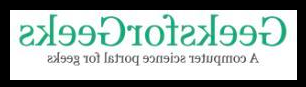Vertical flip: To flip vertically pass image.FLIP_TOP_BOTTOM as keyword

Syntax:

img.transpose(Image.FLIP_TOP_BOTTOM)

Example:

## Python

 `from` `PIL ``import` `Image`  `img ``=` `Image.``open``(``'geek.jpg'``)` `# flip vertical``flip_img ``=` `img.transpose(Image.FLIP_TOP_BOTTOM)` `flip_img.show()`

Output :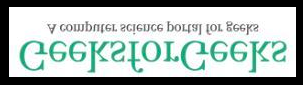## Rotating Images

Image rotation is done by specific angles and for that again specific keywords need to passed as discussed below:

Rotate by 90 degrees: The keyword used for this is Image.ROTATE_90

Syntax :

img.transpose(Image.ROTATE_90)

Example:

## Python

 `from` `PIL ``import` `Image`  `img ``=` `Image.``open``(``'geek.jpg'``)` `# rotate by 90 degrees``rot_img ``=` `img.transpose(Image.ROTATE_90)` `rot_img.show()`

Output :Rotate by 180 degrees: To rotate by 180 degrees the keyword used is Image.ROTATE_180

Syntax :

img.transpose(Image.ROTATE_180)

Example:

## Python

 `from` `PIL ``import` `Image`  `img ``=` `Image.``open``(``'geek.jpg'``)` `# rotate by 180 degrees``rot_img ``=` `img.transpose(Image.ROTATE_180)` `rot_img.show()`

Output: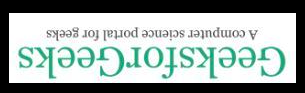Rotate by 270 degrees: To rotate by 270 degrees the keyword used is Image.ROTATE_270

Syntax :

img.transpose(Image.ROTATE_270)

Example:

## Python

 `from` `PIL ``import` `Image`  `img ``=` `Image.``open``(``'geek.jpg'``)` `# rotate by 270 degrees``rot_img ``=` `img.transpose(Image.ROTATE_270)` `rot_img.show()`

Output:My Personal Notes arrow_drop_up